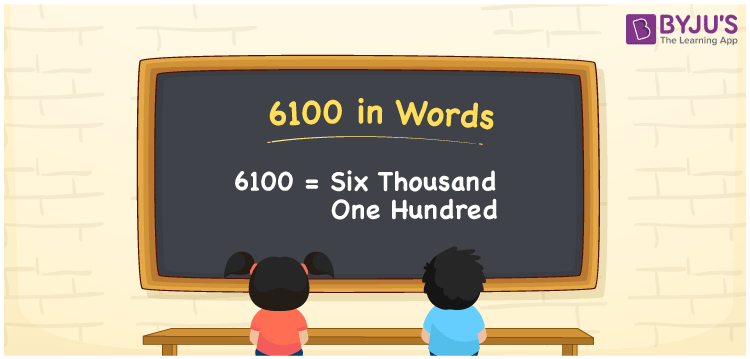# 6100 in Words

6100 in words is written as Six Thousand One Hundred. For example, if you purchased roller skates worth Rs.6100, you can say, “I purchased roller skates worth Rupees Six Thousand One Hundred”. We know that 6100 is a cardinal number. In this article, students will learn the conversion of 6100 into words.

 6100 in Words Six Thousand One Hundred Six Thousand One Hundred in numerical form 6100

## 6100 in English Words

We know that to represent numbers in words, the English alphabet is used. Therefore, 6100 in English words is written as Six Thousand One Hundred.## How to Write 6100 in Words?

6100 in words can be written easily using a place value chart. So, let us make a chart with four columns as 6100 is a four-digit number.

 Thousands Hundreds Tens Ones 6 1 0 0

Therefore, we can write the expanded form as:

6 x Thousand + 1 x Hundred + 0 x Ten + 0 x One

= 6 x 1000 + 1 x 100 + 0 x 10 + 0 x 1

= 6000 + 100 + 0 + 0

= 6000 + 100

= 6100

= Six Thousand One Hundred

Hence, 6100 in words is written as Six Thousand One Hundred.

Interesting way of writing 6100 in words

6 = Six

61 = Sixty-One

610 = Six Hundred and Ten

6100 = Six Thousand One Hundred

Thus, the word form of the number 6100 is Six Thousand One Hundred.

6100 is a natural number that is the successor of 6099 and the predecessor of 6101

• 6100 in words – Six Thousand One Hundred
• Is 6100 an odd number? – No
• Is 6100 an even number? – Yes
• Is 6100 a perfect square number? – No
• Is 6100 a perfect cube number? – No
• Is 6100 a prime number? – No
• Is 6100 a composite number? – Yes

## Frequently Asked Questions on 6100 in Words

Q1

### Write 6100 in English words.

6100 in English words is written as Six Thousand One Hundred.
Q2

### What is the value of 5000 plus 1100?

5000 + 1100 = 6100 Hence, the value of 5000 plus 1100 is 6100.
Q3

### 6100 is a prime number. True or False.

False, 6100 is not a prime number.